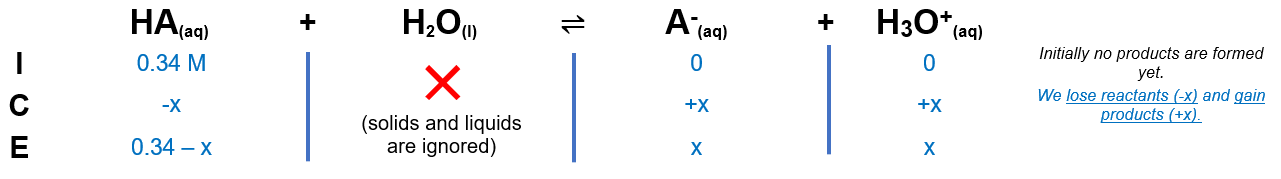# Problem: What is the pH of a 0.34 M solution of a weak acid which has a pKa of 5.40?A. 2.9B. 3.9C. 2.0D. 1.7E. 1.6

###### FREE Expert Solution

We’re being asked to calculate the pH of a 0.34 M weak acid with pKa of 5.40.

Remember that weak acids partially dissociate in water and that acids donate H+ to the base (water in this case). The dissociation of HA is as follows:

HA(aq) + H2O(l)  H3O+(aq) + A(aq)

From this, we can construct an ICE table. Remember that liquids are ignored in the ICE table.The Ka expression for HA is:

$\overline{){{\mathbf{K}}}_{{\mathbf{a}}}{\mathbf{=}}\frac{\mathbf{products}}{\mathbf{reactants}}{\mathbf{=}}\frac{\mathbf{\left[}{\mathbf{H}}_{\mathbf{3}}{\mathbf{O}}^{\mathbf{+}}\mathbf{\right]}\mathbf{\left[}{\mathbf{A}}^{\mathbf{-}}\mathbf{\right]}}{\mathbf{\left[}\mathbf{HA}\mathbf{\right]}}}$

Note that each concentration is raised by the stoichiometric coefficient: [HA], [H3O+] and [A] are raised to 1.

97% (311 ratings)###### Problem Details

What is the pH of a 0.34 M solution of a weak acid which has a pKa of 5.40?

A. 2.9

B. 3.9

C. 2.0

D. 1.7

E. 1.6